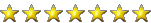# A crack in the standard model - leptoquarks

#### twilyth

#####In a nutshell, there is a rule in the std model called lepton universality which states that "all leptons should be treated equally by the weak interaction, the fundamental force responsible for radioactive decay." So if you have an interaction that creates a W boson, one of two bosons that mediate the weak nuclear force, then when it decays into electron, muon or tau leptons, each should be produced in equal amounts.

However it now appears that tau leptons (the heaviest) are favored in this decay. This isn't a true discovery yet since the significance of the data is only 4 rather than the normal 5 sigma (5 std deviations). But it looks like this is not a fluke and if so, it presents some issues for the std. model.

If the discrepancy is real, rather than a statistical fluke, researchers will then face the tough challenge of figuring out what it means. “This effect is really not the kind that most physicists would have expected,” Ligeti says. “It is not easy to accommodate in the most popular models. In that sense it is quite surprising.”

For instance, the darling of so-called “new physics,” or beyond-the-Standard-Model, ideas—supersymmetry—does not usually predict an effect quite like this. Supersymmetry posits a host of undiscovered particles to mirror the ones already known. Yet none of its predicted particles easily produce this kind of violation of lepton universality. “I don’t think at this point we can say that this points to supersymmetry,” says Hassan Jawahery, a physicist at the University of Maryland and a member of the LHCb collaboration, “but it doesn’t necessarily violate supersymmetry.”

Yet if the signal is real, then some kind of new particle is probably implicated. In all B meson decays, at one point a heavier “virtual” particle is created and then quickly disappears—a strange phenomenon allowed by quantum mechanics. In the Standard Model this virtual particle is always a W boson (a particle that carries the weak force), which interacts equally with all leptons. But if the virtual particle were something more exotic that interacts with each lepton differently, depending on its mass, then more taus could be created at the end because taus are the heaviest leptons (and thus might interact more strongly with the virtual particle).

•Norton